Popularity
6.6
Growing
Activity
0.0
Declining
1,405
75
380

Programming language: Java
Tags: Science     Projects

# XChart alternatives and similar libraries

Based on the "Science" category.
Alternatively, view XChart alternatives based on common mentions on social networks and blogs.

• ### JFreeChart

A 2D chart library for Java applications (JavaFX, Swing or server-side).
• ### #<Sawyer::Resource:0x0000563f68549608>

A JavaFX library containing tiles that can be used for dashboards.
• ### Write Clean Java Code. Always.

Sonar helps you commit clean code every time. With over 600 unique rules to find Java bugs, code smells & vulnerabilities, Sonar finds the issues while you focus on the work.
• ### BioJava

:book::microscope::coffee: BioJava is an open-source project dedicated to providing a Java library for processing biological data.
• ### #<Sawyer::Resource:0x0000563f671eac38>

A JavaFX library for Gauges
• ### Charts

A JavaFX library that contains different kind of charts
• ### ChartFx

A scientific charting library focused on performance optimised real-time data visualisation at 25 Hz update rates for data sets with a few 10 thousand up to 5 million data points.
• ### Chart-FX

A scientific charting library focused on performance optimised real-time data visualisation at 25 Hz update rates for data sets with a few 10 thousand up to 5 million data points. [Moved to: https://github.com/fair-acc/chart-fx]
• ### LogicNG

The Next Generation Logic Library
• ### Orson-Charts

A 3D chart library for Java applications (JavaFX, Swing or server-side).

Do you think we are missing an alternative of XChart or a related project?

##XChart

XChart is a light weight Java library for plotting data.

## Description

XChart is a light-weight and convenient library for plotting data designed to go from data to chart in the least amount of time possible and to take the guess-work out of customizing the chart style.

## Simplest Example

Create a `XYChart` instance via `QuickChart`, add a series of data to it, and either display it or save it as a bitmap.

``````
double[] xData = new double[] { 0.0, 1.0, 2.0 };
double[] yData = new double[] { 2.0, 1.0, 0.0 };

// Create Chart
XYChart chart = QuickChart.getChart("Sample Chart", "X", "Y", "y(x)", xData, yData);

// Show it
new SwingWrapper(chart).displayChart();

// Save it
BitmapEncoder.saveBitmap(chart, "./Sample_Chart", BitmapFormat.PNG);

// or save it in high-res
BitmapEncoder.saveBitmapWithDPI(chart, "./Sample_Chart_300_DPI", BitmapFormat.PNG, 300);
``````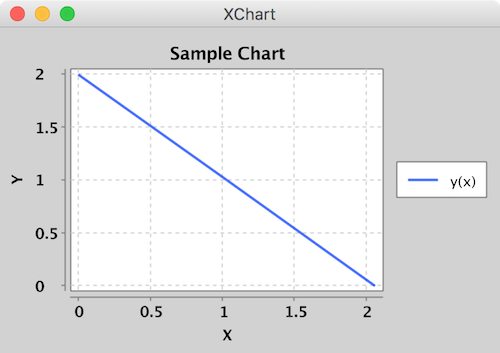## Intermediate Example

Create a `XYChart` via a `XYChartBuilder`, style chart, add a series to it, style series, and display chart.

``````
// Create Chart
XYChart chart = new XYChartBuilder().width(600).height(500).title("Gaussian Blobs").xAxisTitle("X").yAxisTitle("Y").build();

// Customize Chart
chart.getStyler().setDefaultSeriesRenderStyle(XYSeriesRenderStyle.Scatter);
chart.getStyler().setChartTitleVisible(false);
chart.getStyler().setLegendPosition(LegendPosition.InsideSW);
chart.getStyler().setMarkerSize(16);

// Series
chart.addSeries("Gaussian Blob 1", getGaussian(1000, 1, 10), getGaussian(1000, 1, 10));
XYSeries series = chart.addSeries("Gaussian Blob 2", getGaussian(1000, 1, 10), getGaussian(1000, 0, 5));
series.setMarker(SeriesMarkers.DIAMOND);

new SwingWrapper(chart).displayChart();
``````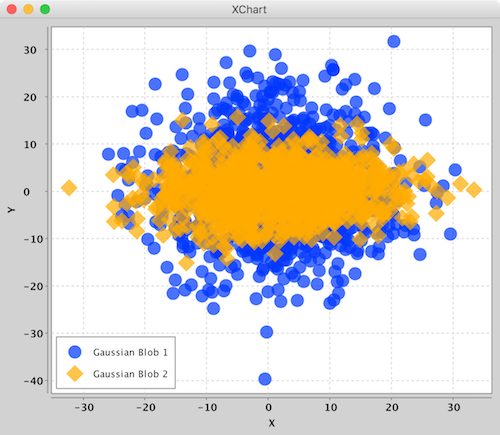Create a `XYChart` via a `XYChartBuilder`, style chart, add a series to it, add chart to `XChartPanel`, embed in Java Swing App, and display GUI.

``````
// Create Chart
final XYChart chart = new XYChartBuilder().width(600).height(400).title("Area Chart").xAxisTitle("X").yAxisTitle("Y").build();

// Customize Chart
chart.getStyler().setLegendPosition(LegendPosition.InsideNE);
chart.getStyler().setDefaultSeriesRenderStyle(XYSeriesRenderStyle.Area);

// Series
chart.addSeries("a", new double[] { 0, 3, 5, 7, 9 }, new double[] { -3, 5, 9, 6, 5 });
chart.addSeries("b", new double[] { 0, 2, 4, 6, 9 }, new double[] { -1, 6, 4, 0, 4 });
chart.addSeries("c", new double[] { 0, 1, 3, 8, 9 }, new double[] { -2, -1, 1, 0, 1 });

// Schedule a job for the event-dispatching thread:
// creating and showing this application's GUI.
javax.swing.SwingUtilities.invokeLater(new Runnable() {

@Override
public void run() {

// Create and set up the window.
JFrame frame = new JFrame("Advanced Example");
frame.setLayout(new BorderLayout());
frame.setDefaultCloseOperation(JFrame.EXIT_ON_CLOSE);

// chart
JPanel chartPanel = new XChartPanel<XYChart>(chart);

// label
JLabel label = new JLabel("Blah blah blah.", SwingConstants.CENTER);

// Display the window.
frame.pack();
frame.setVisible(true);
}
});

``````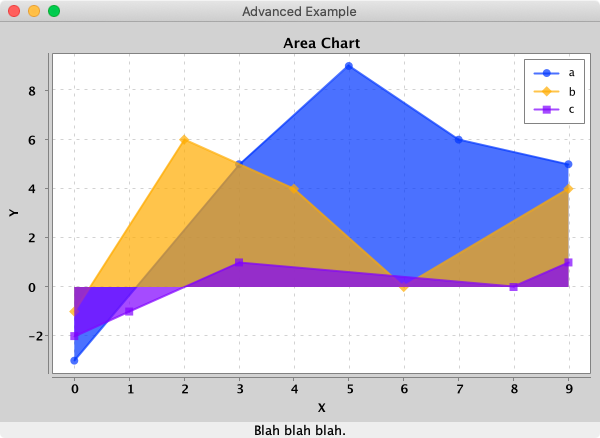To make it real-time, simply call `updateXYSeries` on the `XYChart` instance to update the series data, followed by `revalidate()` and `repaint()` on the `XChartPanel` instance to repaint.

## Features

• [x] No required additional dependencies
• [x] Multiple Y-Axis charts
• [x] Line charts
• [x] Step charts
• [x] Scatter charts
• [x] Area charts
• [x] Step Area charts
• [x] Bar charts
• [x] Histogram charts
• [x] Pie charts
• [x] Donut charts
• [x] Bubble charts
• [x] Stick charts
• [x] Dial charts
• [x] OHLC charts
• [x] Box charts
• [x] Heat maps
• [x] Error bars
• [x] Logarithmic axes
• [x] Number, Date, Bubble and Category X-Axis
• [x] Multiple series
• [x] Tool tips
• [x] Extensive customization
• [x] Themes - XChart, GGPlot2, Matlab
• [x] Right-click, Save-As...
• [x] User-defined axes range
• [x] Definable legend placement
• [x] CSV import and export
• [x] High resolution chart export
• [x] Export as PNG, JPG, BMP, GIF with custom DPI setting
• [x] Export SVG, EPS using optional `de.erichseifert.vectorgraphics2d` library
• [x] Export PDF using optional `pdfbox-graphics2d` library
• [x] Real-time charts
• [x] Java 8 and up

## Chart Types

Currently, there are 5 major chart types. Each type has its corresponding `ChartBuilder`, `Styler` and `Series`.

Chart Type Builder Styler Series Allowed Data Types Default Series Render Style
XYChart XYChartBuilder XYStyler XYSeries Number, Date Line
CategoryChart CategoryChartBuilder CategoryStyler CategorySeries Number, Date, String Bar
PieChart PieChartBuilder PieStyler PieSeries String Pie
BubbleChart BubbleChartBuilder BubbleStyler BubbleSeries Number, Date Round
DialChart DialChartBuilder DialStyler DialSeries double Round
OHLCChart OHLCChartBuilder OHLCStyler OHLCSeries OHLC with Date Candle
BoxChart BoxChartBuilder BoxStyler BoxSeries Number, Date, String Box
HeatMapChart HeatMapChartBuilder HeatMapStyler HeatMapSeries Number, Date, String --

The different Stylers contain chart styling methods specific to the corresponding chart type as well as common styling methods common across all chart types.

### XYChart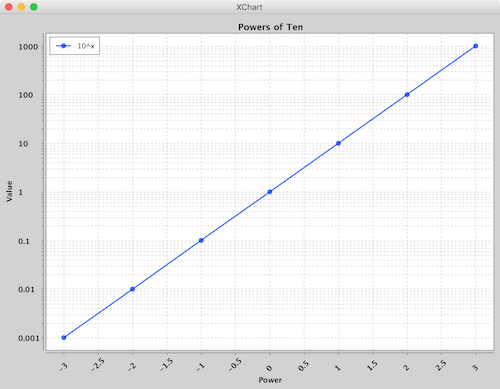`XYChart` charts take Date or Number data types for the X-Axis and Number data types for the Y-Axis. For both axes, the tick marks are auto generated to span the range and domain of the data in evenly-spaced intervals.

Series render styles include: `Line`, `Scatter`, `Area`, `Step` and `StepArea`.

### CategoryChart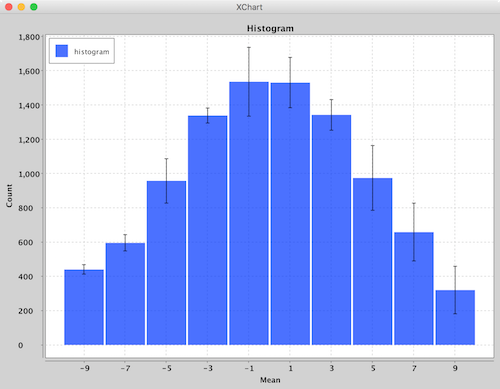`CategoryChart` charts take Date, Number or String data types for the X-Axis and Number data types for the Y-Axis. For the X-Axis, each category is given its own tick mark.

Series render styles include: `Bar`, `Line`, `Scatter`, `Area` and `Stick`.

### PieChart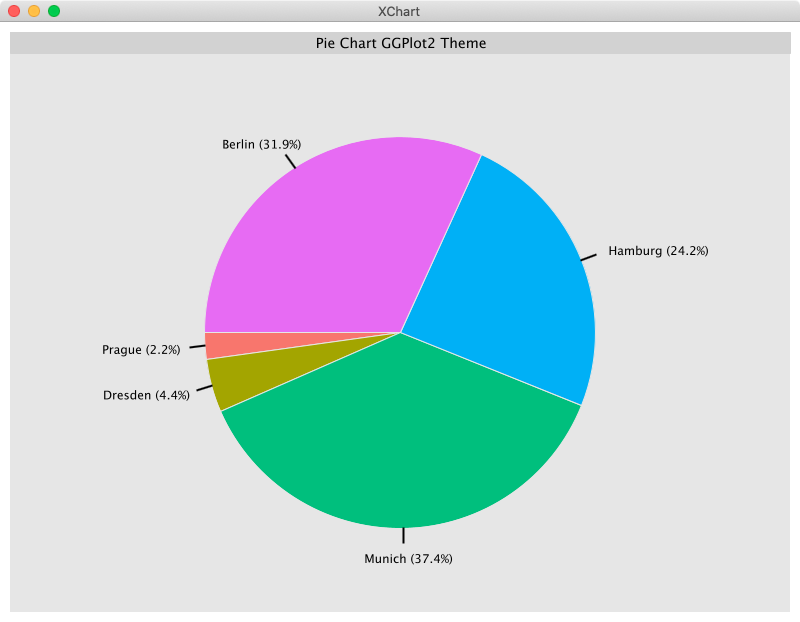`PieChart` charts take String data types for the pie slice name and Number data types for the pie slice value.

Series render styles include: `Pie` and `Donut`.

### BubbleChart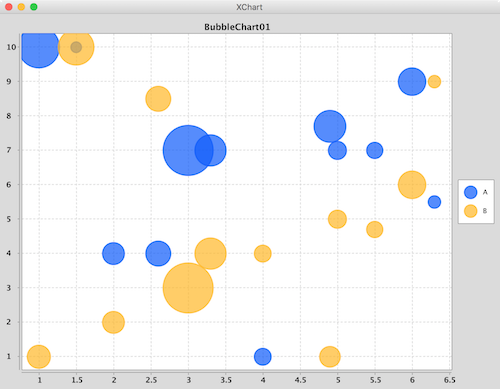`BubbleChart` charts take Date or Number data types for the X-Axis and Number data types for the Y-Axis and bubble sizes.

Series render styles include: `Round` and in the near future `Square`.

### DialChart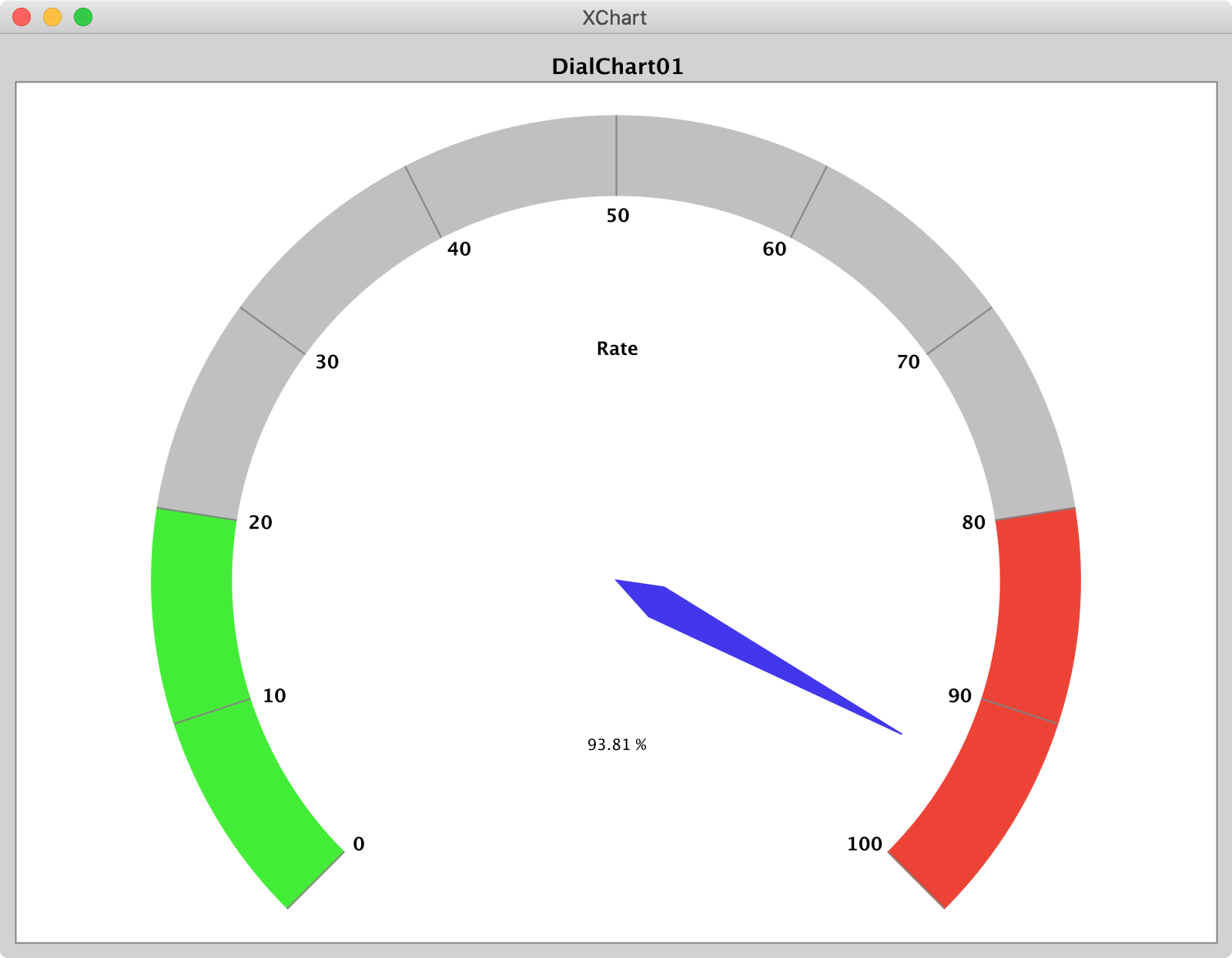`DialChart` charts take a `double` to set the position of the dial pointer and a `String` to set the label. Extensive customization is possible.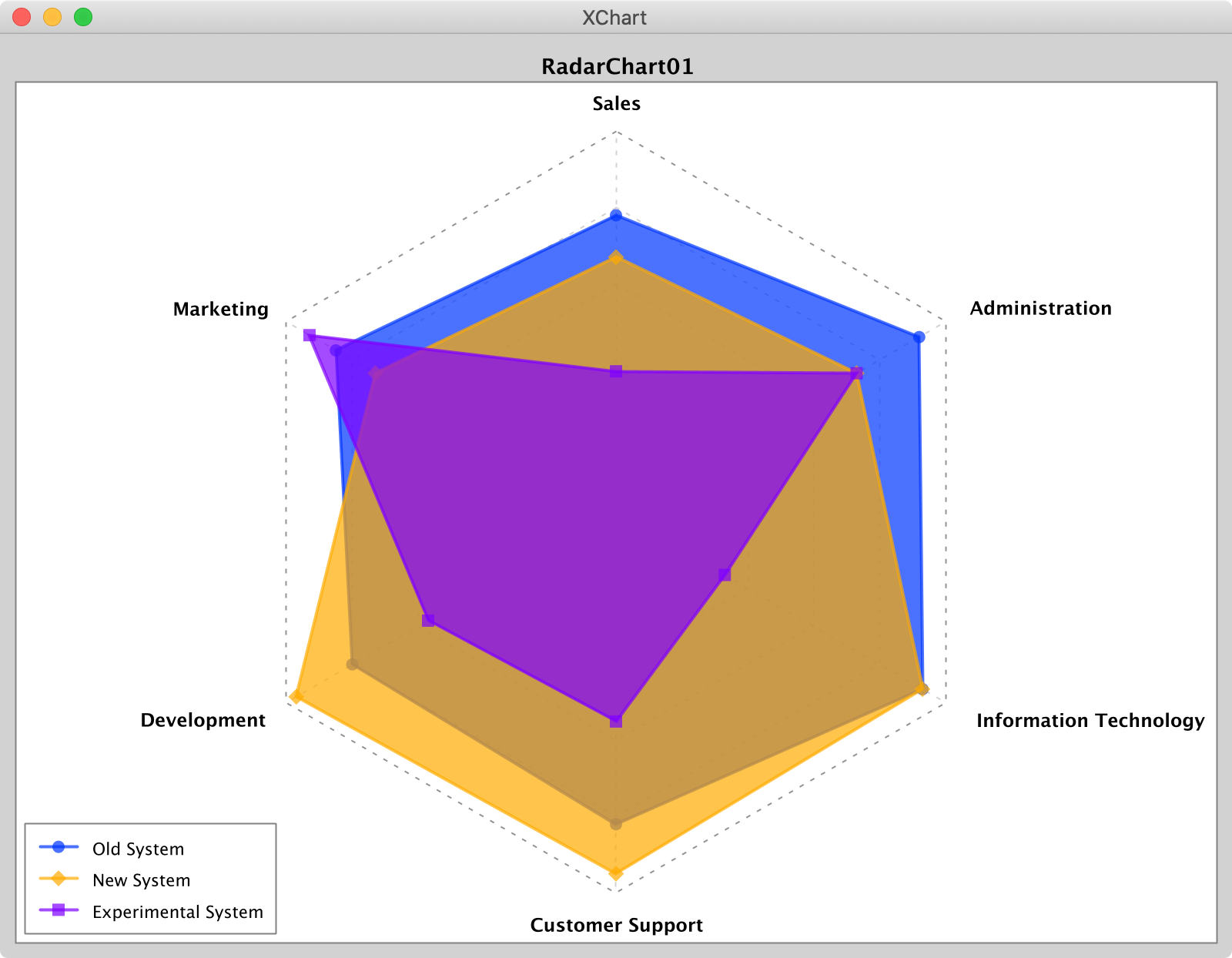`RadarChart` charts take a `double[]` of values between `0.0.` and `1.0` to set the position of the series' data point along each radii. Radii labels, if displayed, are set by passing a `String[]`.

Radar chart render styles are: `Polygon` or `Circle`.

### OHLCChart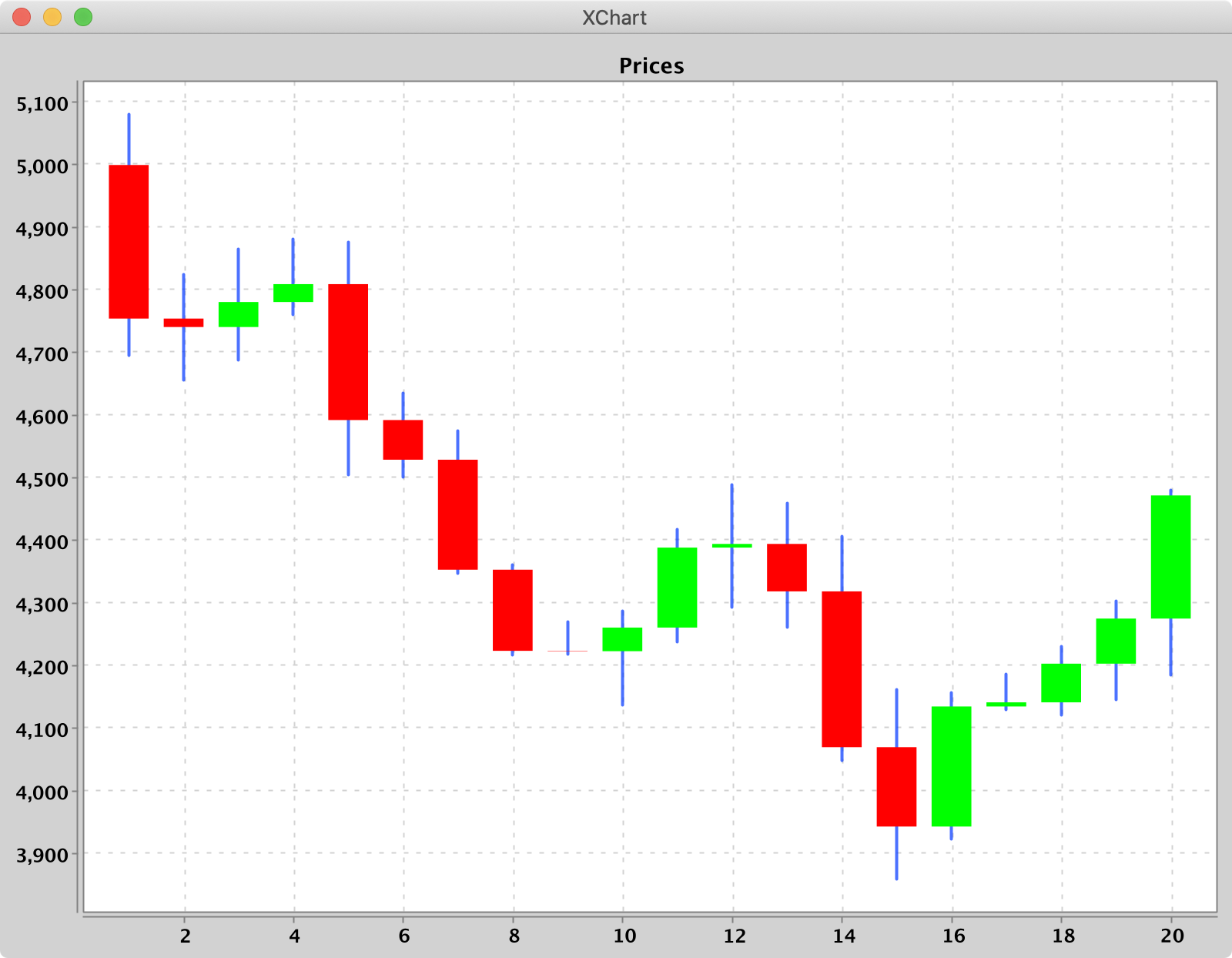`OHLCChart` charts take Date data types for the X-Axis and 4 Number data types for the Y-Axis. For both axes, the tick marks are auto generated to span the range and domain of the data in evenly-spaced intervals.

Series render styles include: `Candle`, `HiLo`.

### BoxChart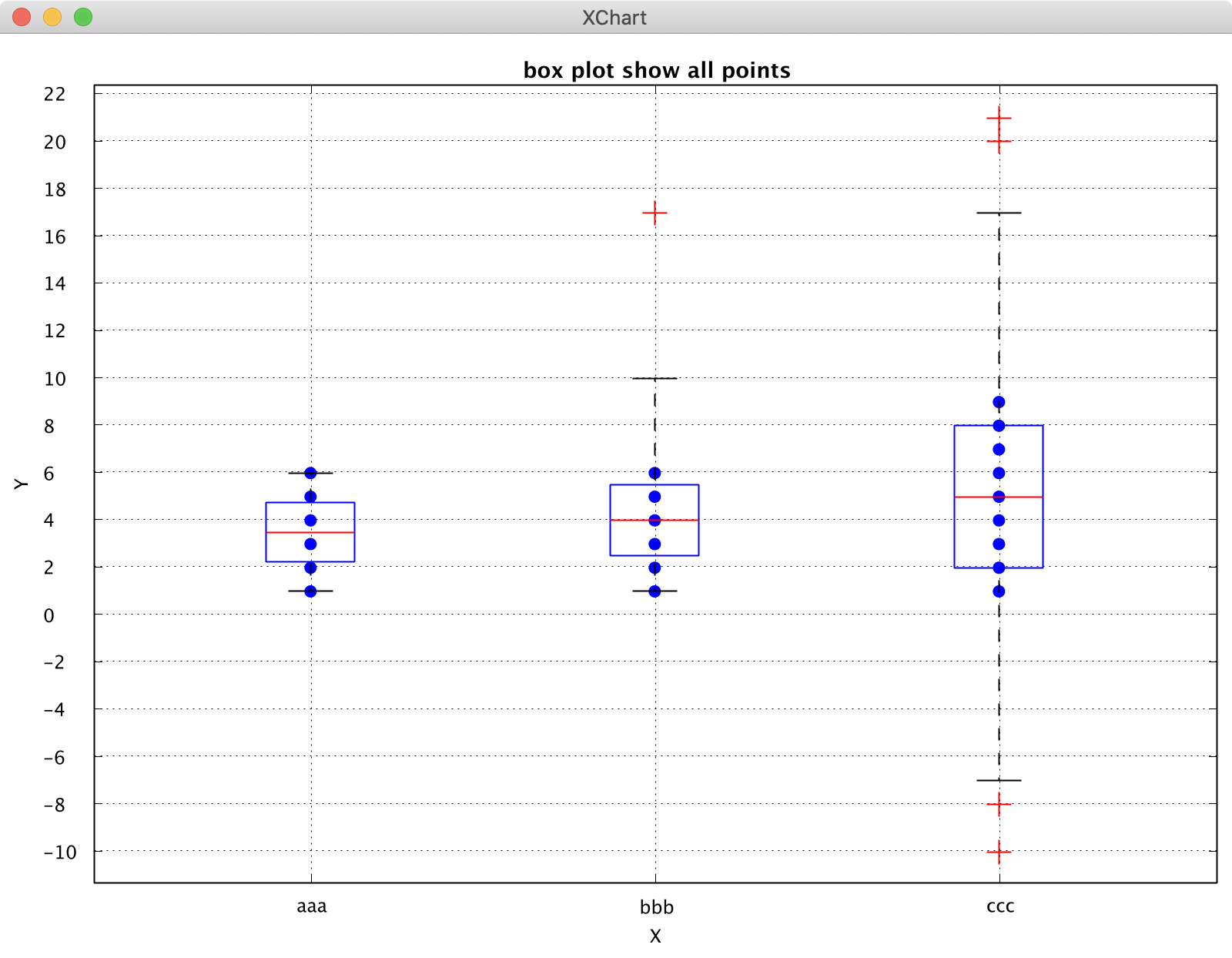`BoxChart` charts take String data (seriesNames) types for the X-Axis and Number data types for the Y-Axis. Each box chart is calculated from the corresponding series yData. Create a BoxChart via a BoxChartBuilder, style chart, add a series to it.

``````// Create Chart
BoxChart chart =
new BoxChartBuilder().title("box plot demo").build();

// Choose a calculation method
chart.getStyler().setBoxplotCalCulationMethod(BoxplotCalCulationMethod.N_LESS_1_PLUS_1);
chart.getStyler().setToolTipsEnabled(true);

// Series
new SwingWrapper<BoxChart>(chart).displayChart();
``````

Four calculation methods for boxplots:

• "N_PLUS_1": determine the position of the quartile, where Qi is = i (n + 1) / 4, where i = 1, 2, and 3. n represents the number of items contained in the sequence. Calculate the corresponding quartile based on location.
• "N_LESS_1": Determine the position of the quartile, where Qi is = i (n-1) / 4, where i = 1, 2, and 3. n represents the number of items contained in the sequence. Calculate the corresponding quartile based on location.
• "NP": Determine the position of the quartile, where Qi is np = (i * n) / 4, where i = 1, 2, and 3. n represents the number of items contained in the sequence. If np is not an integer, Qi = X [np + 1]; If np is an integer, Qi = (X [np] + X [np + 1]) / 2.
• "N_LESS_1_PLUS_1": Determine the position of the quartile, where Qi is = i (n-1) / 4 + 1, where i = 1, 2, 3. n represents the number of items contained in the sequence. Calculate the corresponding quartile based on location.

Interquartile range, IQR = Q3-Q1.

Upper whisker = Q3 + 1.5 * IQR = Q3 + 1.5 * (Q3 - Q1), if Upper whisker is greater than the maximum value of yData, Upper whisker = maximum value of yData.

Lower whisker = Q1 - 1.5 * IQR = Q1 - 1.5 * (Q3 -Q1), if the lower whisker is less than the minimum value of yData, the lower whisker = the minimum value of yData.

E.g:

An example of a set of sequence numbers: 12, 15, 17, 19, 20, 23, 25, 28, 30, 33, 34, 35, 36, 37

• "N_PLUS_1": Q1's position = (14 + 1) /4=3.75, Q1 = 0.25 × third term + 0.75 × fourth term = 0.25 × 17 + 0.75 × 19 = 18.5;
• "N_LESS_1": Q1's location = (14-1) /4=3.25, Q1 = 0.75 × third term + 0.25 × fourth term = 0.75 × 17 + 0.25 × 19 = 17.5;
• "NP": Q1's position = 14 * 0.25 = 3.5, Q1 = 19;
• "N_LESS_1_PLUS_1": Q1's location = (14-1) / 4 + 1 = 4.25 Q1 = 0.75 × the fourth term + 0.25 × the fifth term = 0.75 × 19 + 0.25 × 20 = 19.25.

### HeatMapChart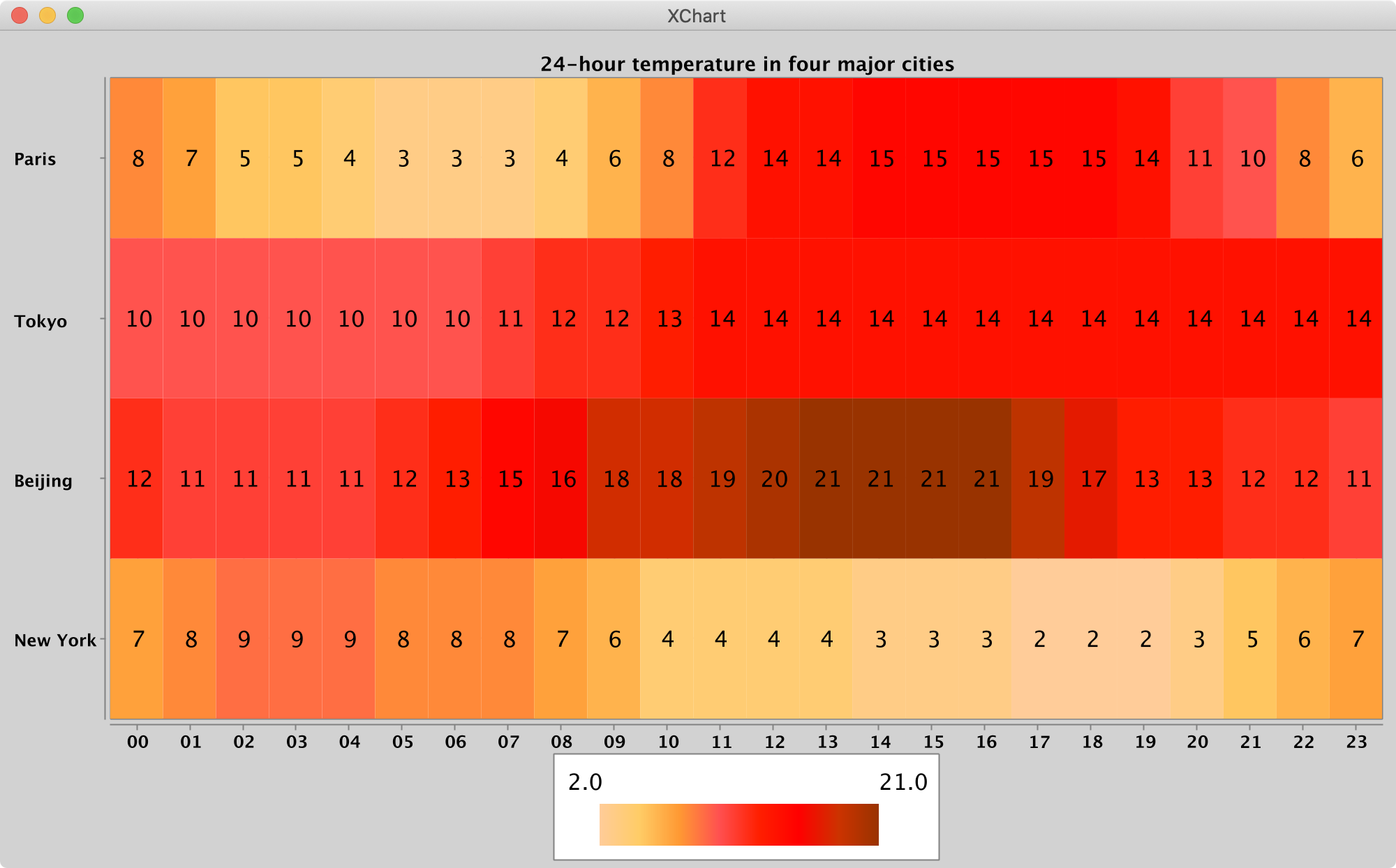`HeatMapChart` take Date, Number or String data types for the X-Axis, Y-Axis.

## Real-time Java Charts using XChart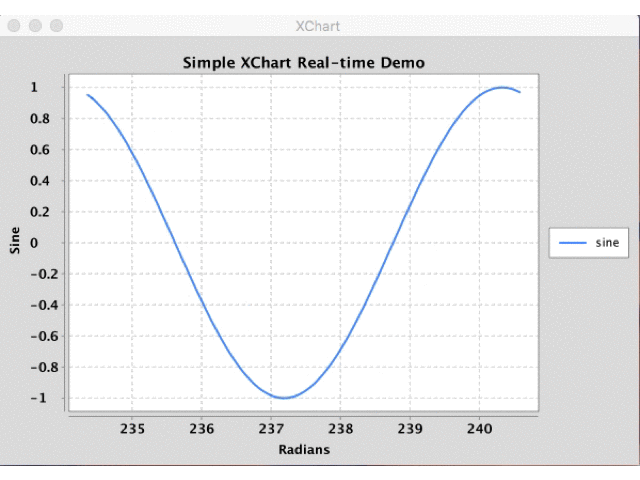Creating real-time charts is as simple as calling `updateXYSeries` for one or more series objects through the `XYChart` instance and triggering a redraw of the `JPanel` containing the chart. This works for all chart types including `XYChart`, `CategoryChart`, `BubbleChart` and `PieChart`, for which example source code can be found here. Examples demonstrate using the `SwingWrapper` with `repaintChart()` method as well as `XChartPanel` with `revalidate()` and `repaint()`.

The following sample code used to generate the above real-time chart can be found here.

``````public class SimpleRealTime {

public static void main(String[] args) throws Exception {

double phase = 0;
double[][] initdata = getSineData(phase);

// Create Chart
final XYChart chart = QuickChart.getChart("Simple XChart Real-time Demo", "Radians", "Sine", "sine", initdata, initdata);

// Show it
final SwingWrapper<XYChart> sw = new SwingWrapper<XYChart>(chart);
sw.displayChart();

while (true) {

phase += 2 * Math.PI * 2 / 20.0;

final double[][] data = getSineData(phase);

javax.swing.SwingUtilities.invokeLater(new Runnable() {

@Override
public void run() {

chart.updateXYSeries("sine", data, data, null);
sw.repaintChart();
}
});
}

}

private static double[][] getSineData(double phase) {

double[] xData = new double;
double[] yData = new double;
for (int i = 0; i < xData.length; i++) {
double radians = phase + (2 * Math.PI / xData.length * i);
}
return new double[][] { xData, yData };
}
}
``````

## Chart Customization

All the styling options can be found in one of two possible places: 1) the Chart's `Styler` or 2) the series' `set` methods. With this chart customization design, all customization options can be quickly "discovered" using an IDE's built in "Content Assist". With centralized styling like this, there is no need to hunt around the entire charting API to find that one customization you're looking for - it's all right in one spot!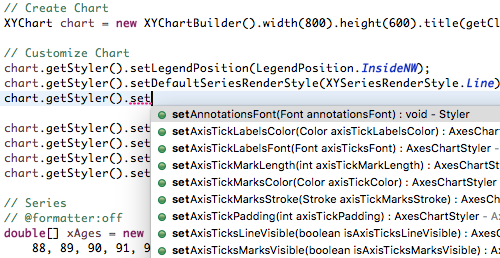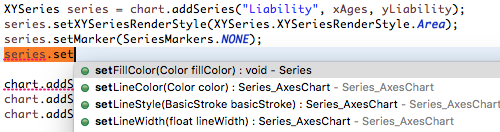### Customizing Axis Tick Labels

XChart automatically creates axis tick labels for chart types with axes.

Default axis tick placement can be altered with `chart.getStyler().setXAxisTickMarkSpacingHint(spacingHint);`.

Default axis label labels can be altered with one of:

``````chart.getStyler().setDatePattern(datePattern)
chart.getStyler().setXAxisDecimalPattern(pattern);
chart.getStyler().setYAxisDecimalPattern(pattern);
``````

You can also create custom axis tick labels with a callback function. In the following example taken from DateChart09, the X-Axis tick labels are generated via a custom lambda function which takes the numerical (double) tick label values and converts them to a `String`.

``````// set custom X-Axis tick labels
LocalDateTime startTime = LocalDateTime.of(2001, Month.JANUARY, 1, 0, 0, 0);
DateTimeFormatter xTickFormatter = DateTimeFormatter.ofPattern("LLL");
chart.getStyler().setxAxisTickLabelsFormattingFunction(x -> startTime.plusDays(x.longValue()).format(xTickFormatter));
``````

In the following example taken from DateChart06, the Y-Axis tick labels are converted to the englich word reprentation of the numbers.

``````chart.getStyler().setyAxisTickLabelsFormattingFunction(x -> NumberWordConverter.convert(x.intValue()));
``````

### Multiple Axes

XChart has multiple y axes feature. Y offset is calculated according to the Y-Axis the series configured. Max `y` value in this axis is calculated according to the series on this axis only. To set the y group:

``````series.setYAxisGroup(axisGroup);
``````

To manually change max/min of axis group:

``````((AxesChartStyler) chart.getStyler()).setYAxisMax(axisGroup, 200.0);
``````

Axis can be drawn on the left (default) or on the right of the chart:

``````chart.getStyler().setYAxisGroupPosition(axisGroup, Styler.YAxisPosition.Right);
``````

To set the Y axes titles:

``````chart.setYAxisGroupTitle(0, "A");
chart.setYAxisGroupTitle(1, "B");
``````

### Zooming In

For the `XYChart` chart type, zooming in is possible on an `XChartPanel` via select-dragging over a range on the X-Axis. Reverting out of the zoom can be accomplished by double-clicking on the chart or by clicking on the "reset" button, which can be posotioned as desired.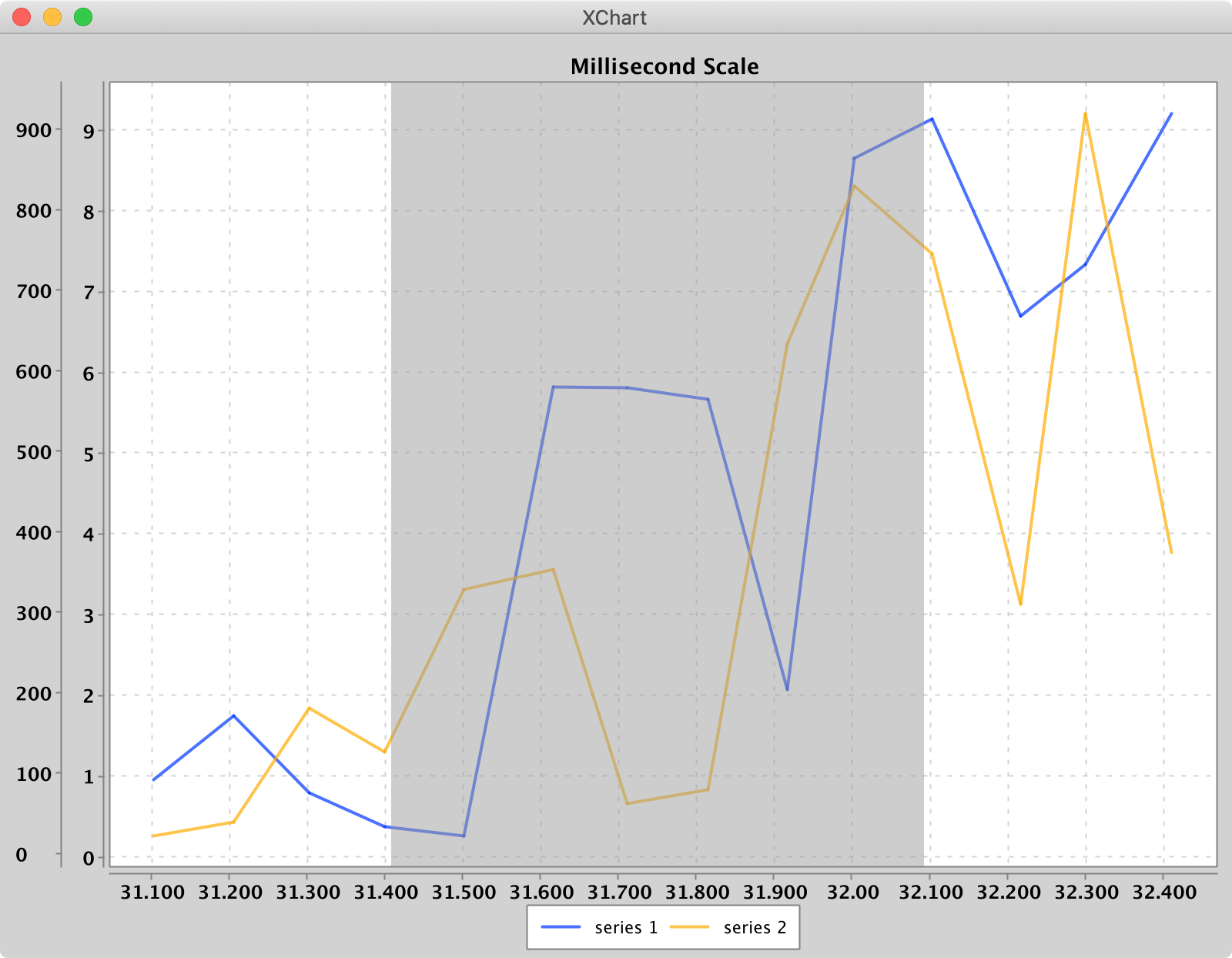The following example zoom style options show which are available:

``````chart.getStyler().setZoomEnabled(true);
chart.getStyler().setZoomResetButtomPosition(Styler.CardinalPosition.InsideS);
chart.getStyler().setZoomResetByDoubleClick(false);
chart.getStyler().setZoomResetByButton(true);
chart.getStyler().setZoomSelectionColor(new Color(0,0 , 192, 128));
``````

A working example can be found at DateChart01.

### Chart Annotations

For all chart types, one or more chart annotations can be super-imposed on top of the chart. The following types of annotatins are available:

• AnnotationLine
• AnnotationImage
• AnnotationText
• AnnotationTextPanel

The following is a chart with four `AnnotationLine`s, one `AnnotationImage` and one `AnnotationText`: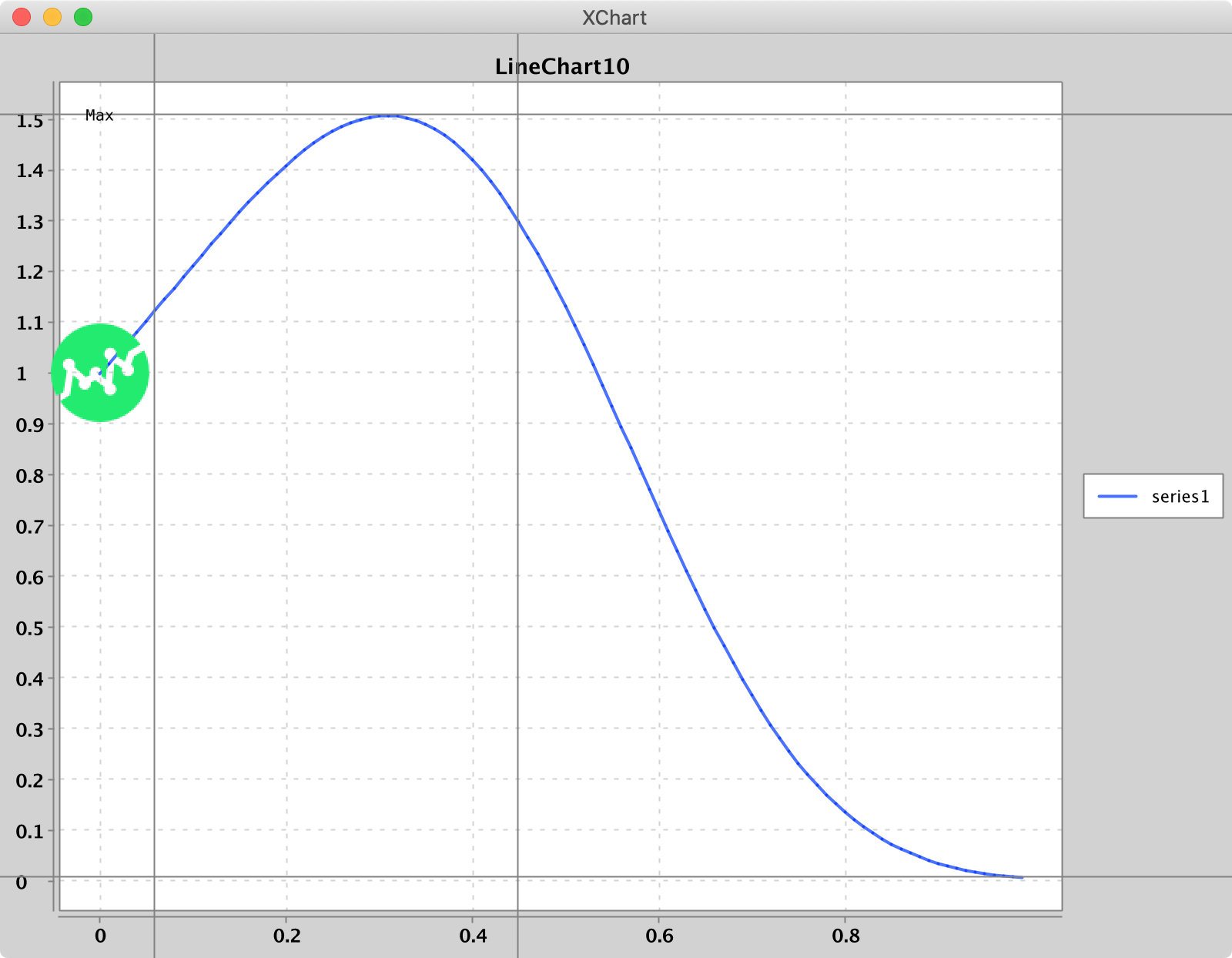Positioning is relative to the bottom-left corner of the chart and to the center of the `AnnotationImage` or `AnnotationText`.

The following example `AnnotationLine` and `AnnotationText` styling parameters show which are available:

``````chart.getStyler().setAnnotationLineColor(Color.GREEN);
chart.getStyler().setAnnotationLineStroke(new BasicStroke(3.0f));
chart.getStyler().setAnnotationTextFont(new Font(Font.MONOSPACED, Font.ITALIC, 8));
chart.getStyler().setAnnotationTextFontColor(Color.BLUE);
``````

A working example can be found at LineChart10.

The following is a chart with three `AnnotationTextPanel`s: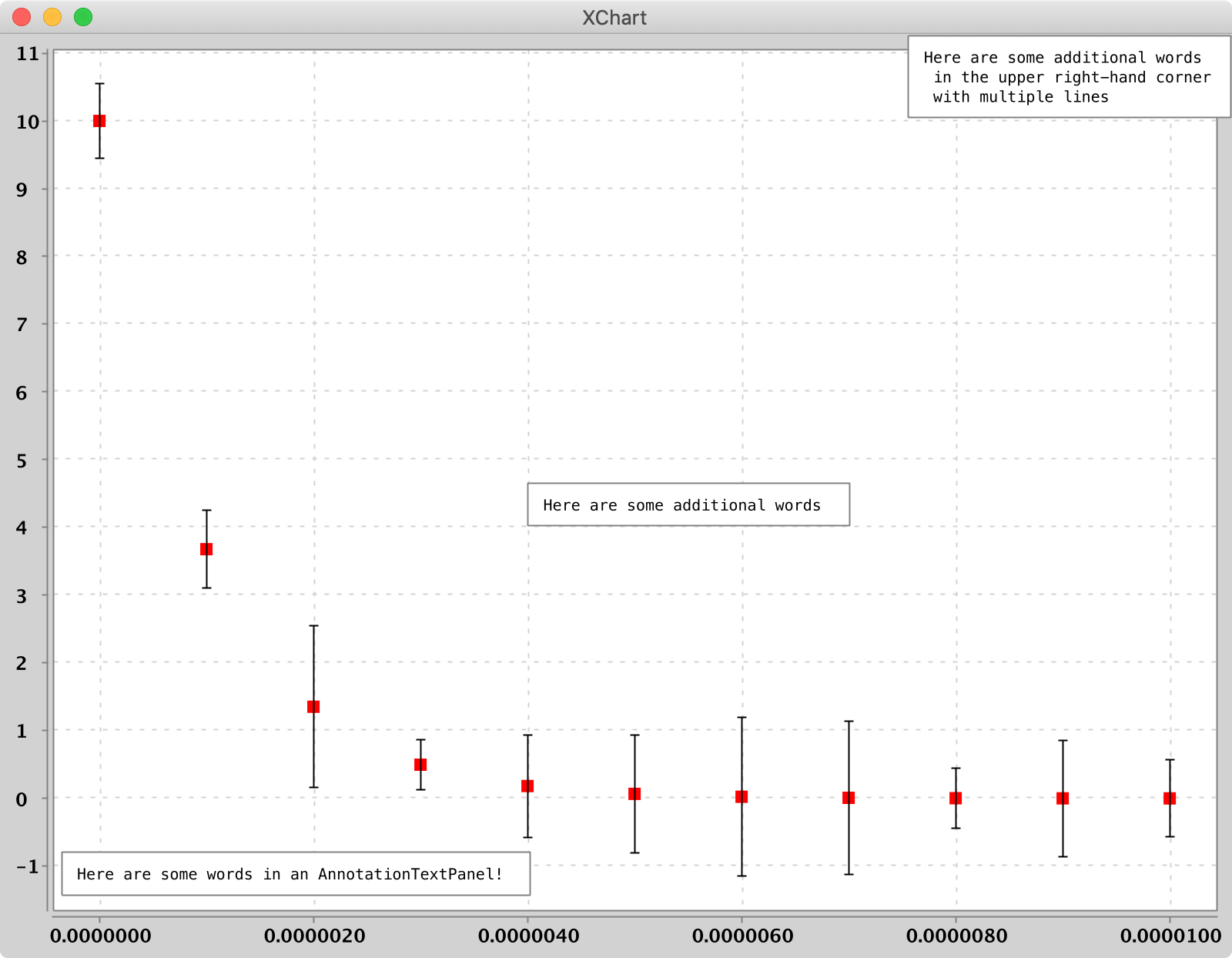Positioning is relative to the bottom-left corner of the chart and to the bottom-left corner of the `AnnotationTextPanel`.

The following example `AnnotationTextPanel` styling parameters show which are available:

``````chart.getStyler().setAnnotationTextPanelPadding(20);
chart.getStyler().setAnnotationTextPanelFont(new Font("Verdana", Font.BOLD, 12));
chart.getStyler().setAnnotationTextPanelBackgroundColor(Color.RED);
chart.getStyler().setAnnotationTextPanelBorderColor(Color.BLUE);
chart.getStyler().setAnnotationTextPanelFontColor(Color.GREEN);
``````

A working example can be found at ScatterChart04.

### Tool Tips

For all chart types, tool tips can be activated on an `XChartPanel` via

``````chart.getStyler().setToolTipsEnabled(true);
``````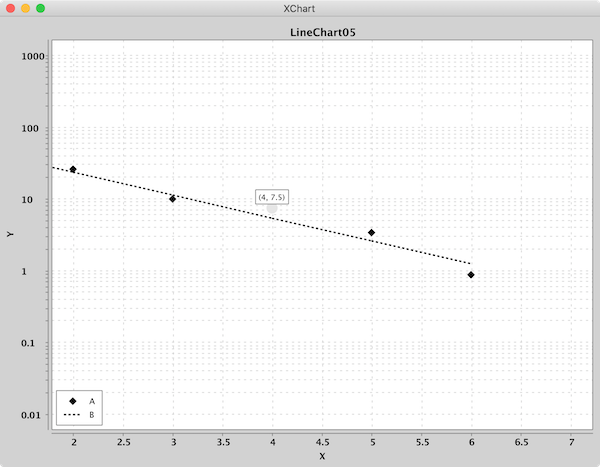The following example tooltip options show which are available:

``````chart.getStyler().setToolTipsEnabled(true);
chart.getStyler(). setToolTipsAlwaysVisible(true);
chart.getStyler().setToolTipFont( new Font("Verdana", Font.BOLD, 12));
chart.getStyler().setToolTipHighlightColor(Color.CYAN);
chart.getStyler().setToolTipBorderColor(Color.BLACK);
chart.getStyler(). setToolTipBackgroundColor(Color.LIGHT_GRAY);
chart.getStyler().setToolTipType(Styler.ToolTipType.xAndYLabels);
``````

A working example can be found at LineChart05.

### Cursor

For the `XYChart` chart type, it is possible to add an interactive cursor on an `XChartPanel` via

``````chart.getStyler().setCursorEnabled(true);
``````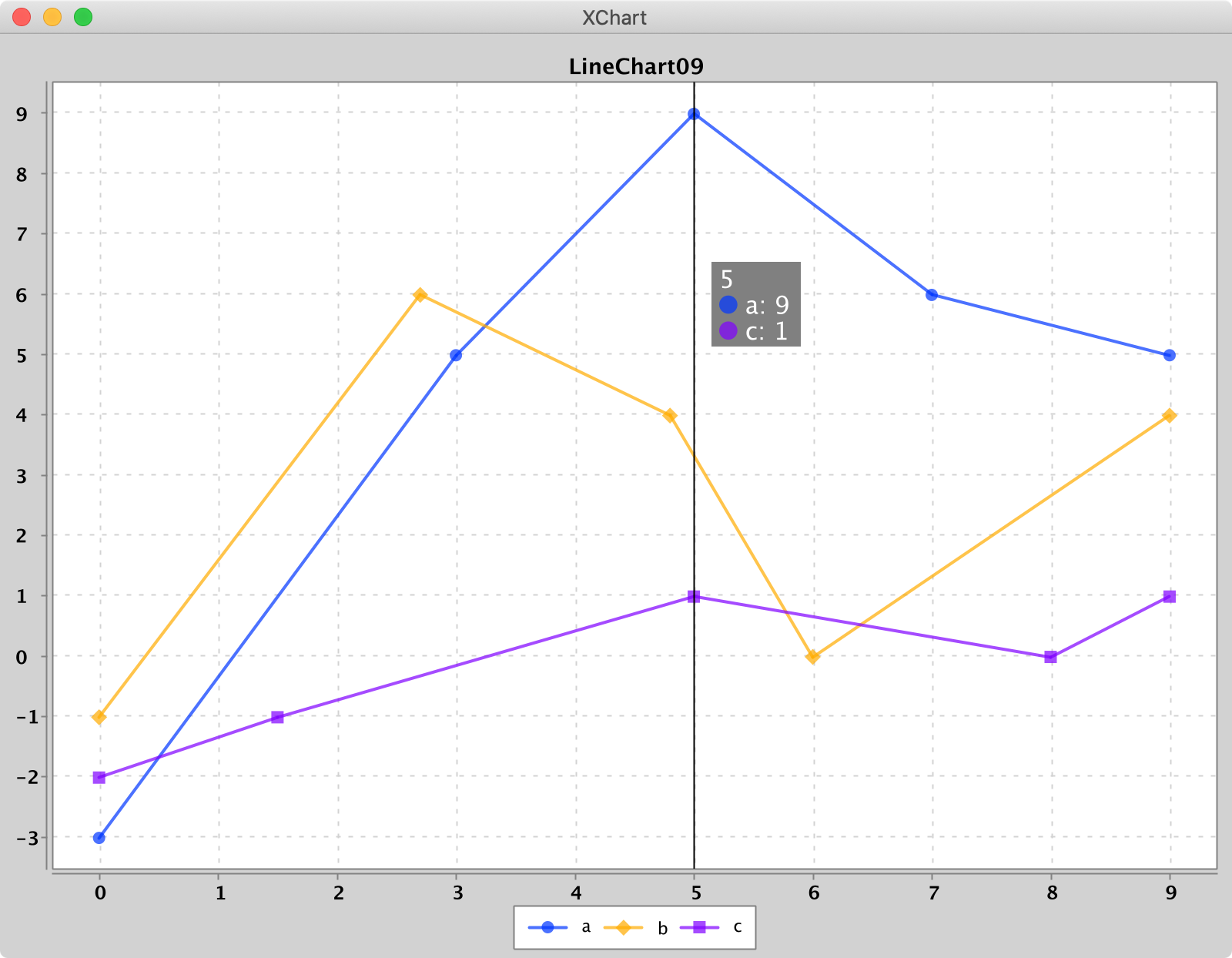The following example cursor options show which are available:

``````chart.getStyler().setCursorEnabled(true);
chart.getStyler().setCursorColor(Color.GREEN);
chart.getStyler().setCursorLineWidth(30f);
chart.getStyler().setCursorFont(new Font("Verdana", Font.BOLD, 12));
chart.getStyler().setCursorFontColor(Color.ORANGE);
chart.getStyler().setCursorBackgroundColor(Color.BLUE);
chart.getStyler().setCustomCursorXDataFormattingFunction(x -> "hello xvalue: " + x);
chart.getStyler().setCustomCursorYDataFormattingFunction(y -> "hello yvalue divided by 2: " + y / 2);
``````

A working example can be found at LineChart09.

## Chart Themes

XChart ships with three different themes: Default `XChart`, `GGPlot2` and `Matlab`. Using a different theme is as simple as setting the Chart's theme with the `theme` method of the `ChartBuilder`.

``````XYChart chart = new XYChartBuilder().width(800).height(600).theme(ChartTheme.Matlab).build();
``````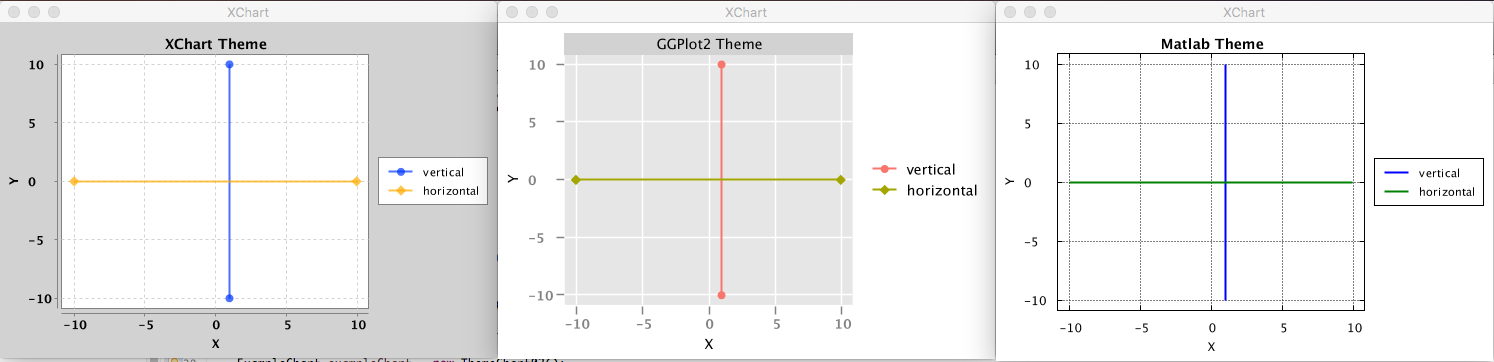## Getting Started

### Maven

The XChart release artifacts are hosted on Maven Central.

``````    <dependency>
<groupId>org.knowm.xchart</groupId>
<artifactId>xchart</artifactId>
<version>3.8.1</version>
</dependency>
``````

``````    <repository>
<id>sonatype-oss-snapshot</id>
<snapshots/>
<url>https://oss.sonatype.org/content/repositories/snapshots</url>
</repository>

<dependency>
<groupId>org.knowm.xchart</groupId>
<artifactId>xchart</artifactId>
<version>3.8.2-SNAPSHOT</version>
</dependency>
``````

### SBT

To use XChart with the Scala Build Tool (SBT) add the following to your build.sbt

``````libraryDependencies += "org.knowm.xchart" % "xchart" % "3.8.0" exclude("de.erichseifert.vectorgraphics2d", "VectorGraphics2D") withSources()
``````

## Building with Maven

#### general

``````mvn clean package
``````

#### Formatting

``````mvn com.coveo:fmt-maven-plugin:format
``````

If you want your IDE to stick to the same format, check out the available configuration plugins:

#### Eclipse

Download `google-java-format-eclipse-plugin_*.jar` and place in `/Applications/Eclipse Java.app/Contents/Eclipse/dropins`. Restart Eclipse. Select the plugin in `Preferences > Java > Code Style > Formatter > Formatter Implementation`.

#### IntelliJ

In the plugins section in IntelliJ search for `google-java-format` and install the plugin. Restart IntelliJ.

## Running Demo - option 1 - using released version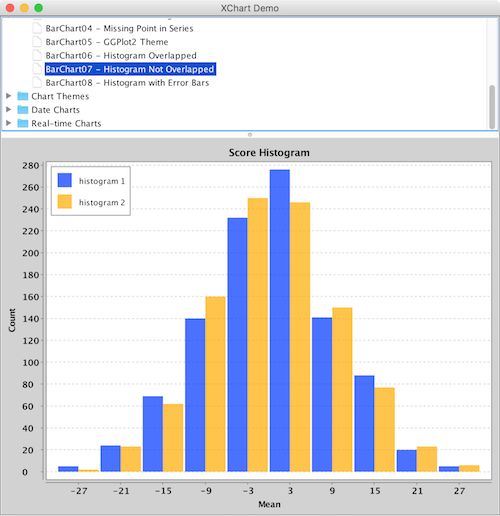• Linux: execute command `java -cp xchart-demo-3.8.0.jar:xchart-3.8.0.jar org.knowm.xchart.demo.XChartDemo`.

• Windows: In the cmd command window, execute the command `java -cp xchart-demo-3.8.0.jar;xchart-3.8.0.jar org.knowm.xchart.demo.XChartDemo`; In the PowerShell command window, execute the command `java -cp "xchart-demo-3.8.0.jar;xchart-3.8.0.jar" org.knowm.xchart.demo.XChartDemo`.

E.g:

``````cd /path/to/xchart-demo/jar/
java -cp xchart-demo-3.8.0.jar:xchart-3.8.0.jar org.knowm.xchart.demo.XChartDemo
``````

## Running Demo - option 2 - building yourself

``````mvn install
mvn exec:java -Djava.awt.headless=false -pl xchart-demo -Dexec.mainClass=org.knowm.xchart.demo.XChartDemo
``````

## Running Demo - option 3 - with tweakable style properties

``````mvn install
mvn exec:java -Djava.awt.headless=false -pl xchart-demo -Dexec.mainClass=org.knowm.xchart.demo.XChartStyleDemo
``````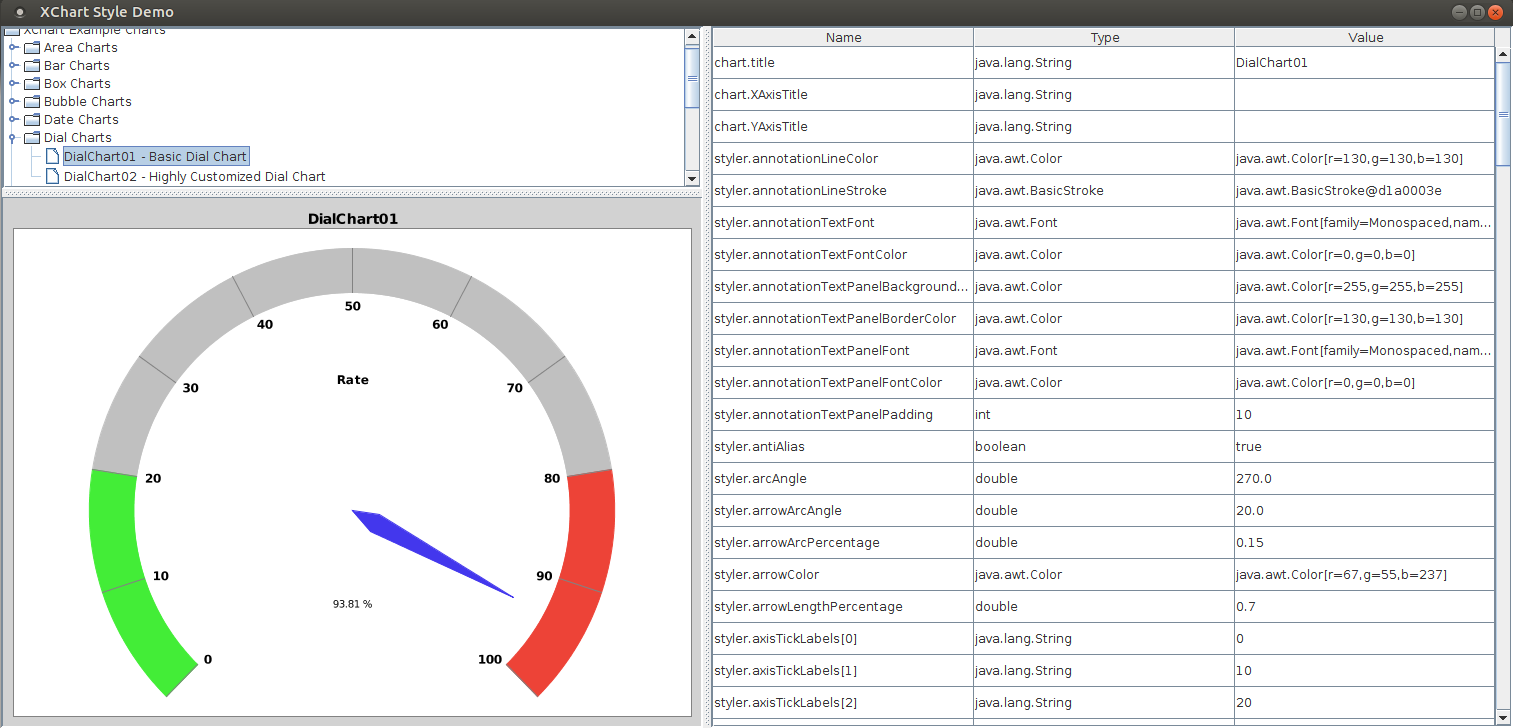## Bugs

Please report any bugs or submit feature requests to XChart's Github issue tracker.